Time: 3 Hours                                                                                                     Max. Marks: 100

NOTE: There are 9 Questions in all.

·      Question 1 is compulsory and carries 20 marks. Answer to Q. 1. must be written in the space provided for it in the answer book supplied and nowhere else.

·      Out of the remaining EIGHT Questions answer any FIVE Questions. Each question carries 16 marks.

·      Any required data not explicitly given, may be suitably assumed and stated.

Q.1       Choose the correct or best alternative in the following:                                         (2x10)

a.       For a conventional FM demodulator, threshold occurs around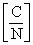equal to

(A)  5 dB                                            (B)  7 dB

(C)    13 dB                                          (D)  40 dB

b.      The frequency band where the attenuation in heavy rain may cause the satellite link to fail is the one using all the frequencies

(A)  above 10 GHz.                             (B)  above 3 GHz.

(C)  between 10GHz & 20 GHz.       (D)  above 6 GHz.

c.   If an earth station antenna has a gain of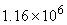, then for a system noise temperature of 79K, the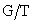ratio of the earth station in dB is

(A)    31.6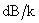(B)  41.6(C) 21.6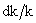(D)  51.6d.   As per ITU-R for the entire geostationary arc, the requirement on the transmit antenna pattern in the range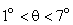from the antenna boresight is

(A)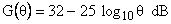(B)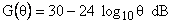(C)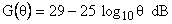(D)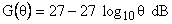e.   The scintillation and absorption effects due to the signal passage through the atmosphere will be

(A)     directly proportional to frequency

(B)     inversely proportional to frequency

(C)     directly proportional to the square of frequency

(D)    inversely proportional to the square of frequency

f.    In the frequency assignment of a typical C-band communications satellite, a typical transponder bandwidth is

(A)     34 MHz                                       (B)  36 MHz

(C)  46 MHz                                       (D)  29 MHz

g.   If the transmission bit rate for an INTELSAT frame that uses QPSK modulation is 120.832 Mb/s, then for a frame efficiency of 0.949, the voice-channel capacity for the frame is

(A)     1792                                            (B)  1782

(C)  1682                                            (D)  1892

h.   If the coding gain for a CDMA receiver is 27.2 dB, then the value of the bit rate for a chip rate of 5 Mcps is

(A)    5 kbps                                         (B) 8.6 kbps

(C) 9.6 kbps                                       (D) 10 kbps

i.    The capacity of a lowpass channel with a usable bandwidth of 3000 Hz and a S/N value of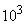at the channel output is

(A)   30 kbps                                        (B) 25 kbps

(C) 40 kbps                                        (D) 35 kbps

j.    Most VSAT systems operate with earth station antenna diameters of

(A)  5 to 7 m                                       (B)  4 to 6 m

(C)  1 to 2 m                                       (D) above 7 m

Answer any FIVE Questions out of EIGHT Questions.

Each question carries 16 marks.

Q.2     a.   With a neat sketch for illustration, define the following:

(i)    Look angles                                 (ii)  Azimuth

(iii)  Elevation                                       (iv) The subsatellite point.

Illustrate by a neat sketch, the geometry of the elevation angle calculation and briefly explain the equations for the calculation of the elevation angle.    (12)

b.   Details of an earth station site and a geostationary satellite in the Indian Ocean operated by Intelsat are given below:

Earth station latitude and longitude :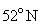and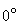Subsatellite point :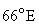(i)                  Can the earth station see the satellite?

(ii)                What is the elevation angle?                                                        (4)

Q.3     a.   What are the factors that determine the weight of a satellite?  Name the component of the satellite communication system which acts as a limiting factor in the capacity and performance of the system.  Usually the uplink design is easier than the downlink in many cases, why?  Explain how the required transmit power can be computed, when the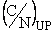ratio is specified for the transponder.  Also, indicate how the received power at the transponder input can be calculated with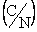UP specified.         (11)

b.   A transponder of a Ku-band satellite has a linear gain of 127 dB and a nominal output power at saturation of 5W.  The gain of satellite’s receiving antenna at 14 GHz is 26 dB on axis and the beam covers a part of Europe.  Calculate the power output of an uplink transmitter so as to ensure an output power of 1W from the satellite transponder at a frequency of 14.45 GHz. The earth station antenna has a gain of 50dB and there is a 1.5dB loss in waveguide run between the transmitter and antenna.  Assume that under clear sky conditions, the atmospheric loss equals 0.5dB and that the earth station is located on the –2dB contour of satellites receiving antenna.  If rain in the path causes attenuation of 7 dB for 0.01% of the year, what output power rating, in watts, is required for the transmitter to guarantee that a one watt output can be obtained from the satellite transponder for 99.99% of the year, if uplink power control is used?  Take the path length as 38500 Km.                                                                                                                   (5)

Q.4     a.   What do you mean by rain rate?  When the rain rate is specified as 0.001 percent, what does it mean?  Define the quantity specific attenuation. (6)

b.   From first principles, derive the equation for rain attenuation in decibels.            (10)

Q.5     a.   In satellite links, for transmission of digital signals, FSK system is rarely used.  Give proper reasoning on the choice to be made and mention the type of frequency modulation that is often preferred.  What do you mean by error free transmission in a digital telephone system?                                       (4)

b.   With reference to TDMA, (i) Explain the function of a unique word correlator. (ii) Derive an expression for the number of digital speech channels that can be transmitted in a TDMA frame shared equally by N earth stations in terms of frame time, guard time, preamble length and TDMA rate.                      (10)

c.   The down link transmission rate in a satellite circuit is 61 Mbps and the required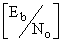at the ground station receiver is 9.5dB.  Calculate the required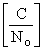.                                                   (2)

Q.6     a.   Write the meaning of the following as used in satellite links:

(i)   Polarisation Isolation                      (ii)   Frequency reuse.                             (6)

b.   Write a note on antennas carried aboard a satellite.                                             (7)

c.  The earth station of a satellite network uses an antenna of 12 m diameter with an efficiency of 65%.  If the satellite’s transmitting frequency is         4 GHz, determine the gain of the earth station antenna.        (3)

Q.7     a.   Distinguish between multiplexing and multiple access.  Comment on the basic problem in any multiple access system.  What do you mean by demand access and hybrid multiple access?        (7)

b.   Define the following with reference to CDMA technique:

(i)  DS-SS CDMA Capacity                (ii)  PN Sequences                                  (4)

c.   Derive the output SNR relation for the bit stream in the receiver of a       DS-SS CDMA system, where there are a number of CDMA signals present at the input to each receiver.                          (5)

Q.8     a.   Distinguish between block and convolutional codes. Explain the Shannon-

Hartley Law for a digital communication link.                                                      (9)

b.   The generator matrix for a (6, 3) block code is given below:(i)                  How many message blocks are of interest?

(ii)                Find the code words for the message blocks:                               (0 1 0), (1 0 0) and (1 1 1).                               (7)

Q.9     a.   Which is the key factor in making the VSAT service both economically attractive to the user and environmentally acceptable to the community?  With a neat illustration, briefly explain the star VSAT network architecture.                                                                                                       (7)

b.   Explain the three network architecture schemes used in satellite systems. What is spoofing?             (9)